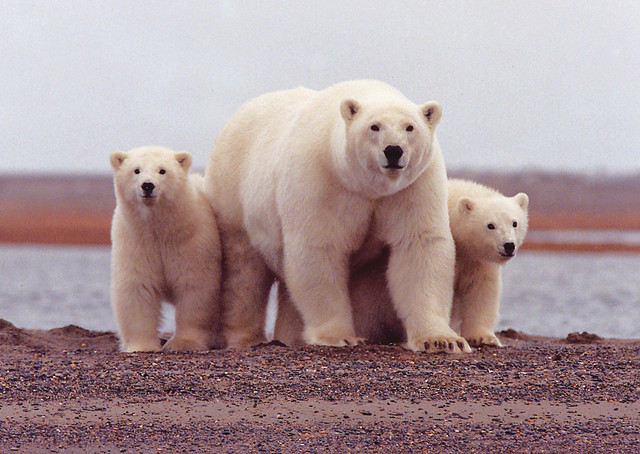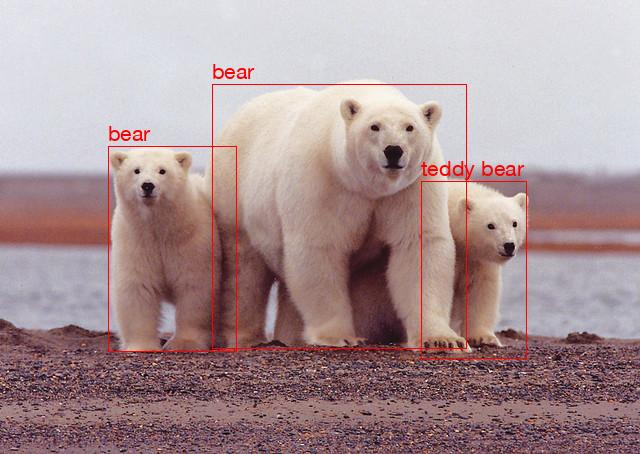# TensorFlow Object Detection in PHP

The ONNX Runtime package makes it easy to run TensorFlow models in PHP. This short tutorial will show you how. It’s based on this tutorial from tf2onnx.

We’ll use SSD Mobilenet, which can detect multiple objects in an image.

First, download the pretrained model from the official TensorFlow Models project and this awesome shot of polar bears.Photo from the U.S. Fish and Wildlife Service

Install tf2onnx

``````pip install tf2onnx
``````

And convert the model to ONNX

``````python -m tf2onnx.convert --opset 10 \
--saved-model ssd_mobilenet_v1_coco_2018_01_28/saved_model \
--output model.onnx
``````

Next, install the ONNX Runtime package

``````composer require ankane/onnxruntime
``````

``````composer exec -- php -r "require 'vendor/autoload.php'; OnnxRuntime\Vendor::check();"
``````

``````\$img = imagecreatefromjpeg('bears.jpg');
``````

Get the pixel values

``````function getPixels(\$img)
{
\$pixels = [];
\$width = imagesx(\$img);
\$height = imagesy(\$img);
for (\$y = 0; \$y < \$height; \$y++) {
\$row = [];
for (\$x = 0; \$x < \$width; \$x++) {
\$rgb = imagecolorat(\$img, \$x, \$y);
\$color = imagecolorsforindex(\$img, \$rgb);
\$row[] = [\$color['red'], \$color['green'], \$color['blue']];
}
\$pixels[] = \$row;
}
return \$pixels;
}

\$pixels = getPixels(\$img);
``````

``````\$model = new OnnxRuntime\Model('model.onnx');
``````

Check the model inputs

``````print_r(\$model->inputs());
``````

The shape is `[-1, -1, -1, 3]`. `-1` indicates any size. `pixels` has the shape `[\$height, \$width, 3]`. The model is designed to process multiple images at once, which is where the final dimension comes from.

Let’s run the model:

``````\$result = \$model->predict(['inputs' => [\$pixels]]);
``````

The model gives us a number of different outputs, like the number of detections, labels, scores, and boxes. Let’s print the results:

``````print_r(\$result['num_detections']);
// 
print_r(\$result['detection_classes']);
// [[23, 23, 88, ...]]
``````

We can see there were three detections, and if we look at the first three elements in the detection classes array, they are the numbers 23, 23, and 88. These correspond to COCO labels. We can look these up and see that 23 is bear and 88 is teddy bear. Mostly right!

With a bit more code, we can apply boxes and labels to the image.

``````\$coco_labels = [
23 => 'bear',
88 => 'teddy bear'
];

function drawBox(&\$img, \$label, \$box)
{
\$width = imagesx(\$img);
\$height = imagesy(\$img);

\$top = round(\$box * \$height);
\$left = round(\$box * \$width);
\$bottom = round(\$box * \$height);
\$right = round(\$box * \$width);

// draw box
\$red = imagecolorallocate(\$img, 255, 0, 0);
imagerectangle(\$img, \$left, \$top, \$right, \$bottom, \$red);

// draw text
\$font = '/System/Library/Fonts/HelveticaNeue.ttc';
imagettftext(\$img, 16, 0, \$left, \$top - 5, \$red, \$font, \$label);
}

foreach (\$result['num_detections'] as \$idx => \$n) {
for (\$i = 0; \$i < \$n; \$i++) {
\$label = intval(\$result['detection_classes'][\$idx][\$i]);
\$label = \$coco_labels[\$label] ?? \$label;
\$box = \$result['detection_boxes'][\$idx][\$i];
drawBox(\$img, \$label, \$box);
}
}

// save image
imagejpeg(\$img, 'labeled.jpg');
``````

And the result:Here’s the complete code. Now go out and try it with your own images!

Published August 24, 2022

You might also enjoy

## Strong Encryption Keys for Rails

All code examples are public domain.
Use them however you’d like (licensed under CC0).